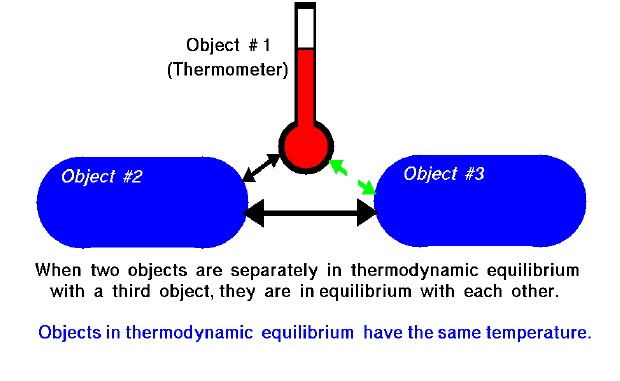# Zeroth Law – Thermal Equilibrium## Thermodynamics

Thermodynamics is a branch of physics which deals with the energy and work of a system. Thermodynamics deals only with the large scale response of a system which we can observe and measure in experiments. There are three principal laws of thermodynamics which are described on separate slides. Each law leads to the definition of thermodynamic properties which help us to understand and predict the operation of a physical system. We will present some simple examples of these laws and properties for a variety of physical systems, although, like the Wright brothers, we are most interested in the thermodynamics of engines. Fortunately, many of the classical examples involve gas dynamics. Unfortunately, the numbering system for the three laws of thermodynamics is a bit confusing. We begin with the zeroth law.

## Zeroth Law

The zeroth law of thermodynamics begins with a simple definition of thermodynamic equilibrium. It is observed that some property of an object, like the pressure in a volume of gas, the length of a metal rod, or the electrical conductivity of a wire, can change when the object is heated or cooled. If two of these objects are brought into physical contact there is initially a change in the property of both objects. Eventually the change in property stops and the objects are said to be in thermal (thermodynamic) equilibrium. Thermodynamic equilibrium leads to the large scale definition of temperature. When two objects are in thermal equilibrium they are said to have the same temperature. During the process of reaching thermal equilibrium, heat, which is a form of energy, is transferred between the objects. The details of the process of reaching thermal equilibrium are described in the first and second laws of thermodynamics.

## Thermal Equilibrium

The zeroth law of thermodynamics is an observation. When two objects are separately in thermodynamic equilibrium with a third object, they are in equilibrium with each other. As an illustration, suppose we have three objects as shown on the slide. Object #1 and object #2 are in physical contact and in thermal equilibrium. Object #2 is also in thermal equilibrium with object #3. There is initially no physical contact between object #1 and object #3. But, if object #1 and object #3 are brought into contact, it is observed that they are in thermal equilibrium. This simple observation allows us to create thermometers. We can calibrate the change in a thermal property (length of a column of mercury) by putting the thermometer in thermal equilibrium with a known physical system. Metric thermometers (Celsius) have the reference points fixed at the freezing and boiling point of pure water. If we then bring the thermometer into thermal equilibrium with any other system (place it under your tongue) we can determine the temperature of the other system by noting the change in the thermal property. Objects in thermodynamic equilibrium have the same temperature.

Provide feedback